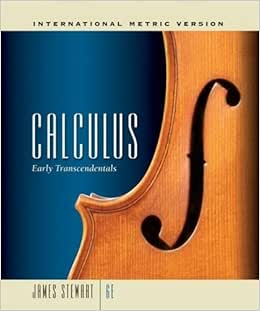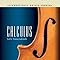\$151.19
& FREE Delivery
Usually dispatched within 3 to 4 days.
Ships from and sold by Near Fine_Books.
##### Other Sellers on Amazon
\$163.18
+ FREE Delivery
Sold by: Book Depository UK
\$178.00
+ FREE Delivery
Sold by: Blackwell UK Ltd
\$213.00
+ FREE DeliveryFlip to back Flip to front
Listen Playing... Paused   You're listening to a sample of the Audible audio edition.See this image

# Calculus : Early Transcendentals, International Metric Edition Hardcover – 21 December 2007

See all formats and editions Hide other formats and editions
 Amazon Price New from Used from
 Hardcover "Please retry" \$151.19 \$151.19 —
Arrives: 11 - 16 Sep

## Product details

• Hardcover: 1312 pages
• Publisher: Brooks/Cole ISE; 6 edition (21 December 2007)
• Language: English
• ISBN-10: 0495382736
• ISBN-13: 978-0495382737
• Product Dimensions: 22.3 x 4.2 x 26 cm
• Boxed-product Weight: 2.4 Kg
• Customer Reviews:

## Product description

### Review

1. Functions and Models. Four Ways to Represent a Function. Mathematical Models: A Catalog of Essential Functions. New Functions from Old Functions. Graphing Calculators and Computers. Exponential Functions. Inverse Functions and Logarithms. Principles of Problem Solving. 2. Limits and Derivatives. The Tangent and Velocity Problems. The Limit of a Function. Calculating Limits Using the Limit Laws. The Precise Definition of a Limit. Continuity. Limits at Infinity; Horizontal Asymptotes. Derivatives and Rates of Change. Writing Project: Early Methods for Finding Tangents. The Derivative as a Function. 3. Differentiation Rules. Derivatives of Polynomials and Exponential Functions. Applied Project: Building a Better Roller Coaster. The Product and Quotient Rules. Derivatives of Trigonometric Functions. The Chain Rule. Applied Project: Where Should a Pilot Start Descent? Implicit Differentiation. Derivatives of Logarithmic Functions. Rates of Change in the Natural and Social Sciences. Exponential Growth and Decay. Related Rates. Linear Approximations and Differentials. Laboratory Project: Taylor Polynomials. Hyperbolic Functions. 4. Applications of Differentiation. Maximum and Minimum Values. Applied Project: The Calculus of Rainbows. The Mean Value Theorem. How Derivatives Affect the Shape of a Graph. Indeterminate Forms and L'Hospital's Rule. Writing Project: The Origins of L'Hospital's Rule. Summary of Curve Sketching. Graphing with Calculus and Calculators. Optimization Problems. Applied Project: The Shape of a Can. Applications to Business and Economics. Newton's Method. Antiderivatives. 5. Integrals. Areas and Distances. The Definite Integral. Discovery Project: Area Functions. The Fundamental Theorem of Calculus. Indefinite Integrals and the Total Change Theorem. Writing Project: Newton, Leibniz, and the Invention of Calculus. The Substitution Rule 6. Applications of Integration. Areas between Curves. Volume. Volumes by Cylindrical Shells. Work. Average Value of a Function. Applied Project: Where to Sit at the Movies. 7. Techniques of Integration. Integration by Parts. Trigonometric Integrals. Trigonometric Substitution. Integration of Rational Functions by Partial Fractions. Strategy for Integration. Integration Using Tables and Computer Algebra Systems. Discovery Project: Patterns in Integrals. Approximate Integration. Improper Integrals. Review. Problems Plus. 8. Further Applications of Integration. Arc Length. Discovery Project: Arc Length Contest. Area of a Surface of Revolution. Discovery Project: Rotating on a Slant. Applications to Physics and Engineering. Applications to Economics and Biology. Probability. Review. Problems Plus. 9. Differential Equations. Modeling with Differential Equations. Direction Fields and Euler's Method. Separable Equations. Applied Project: Which is Faster, Going Up or Coming Down? Exponential Growth and Decay. Applied Project: Calculus and Baseball. The Logistic Equation. Linear Equations. Predator-Prey Systems. Review. Problems Plus. 10. Parametric Equations and Polar Coordinates. Curves Defined by Parametric Equations. Laboratory Project: Families of Hypocycloids. Tangents and Areas. Laboratory Project: Bezier Curves. Arc Length and Surface Area. Polar Coordinates. Areas and Lengths in Polar Coordinates. Conic Sections. Conic Sections in Polar Coordinates. Applied Project: Transfer Orbits. Review. Problems Plus. 11. Infinite Sequences and Series. Sequences. Laboratory Project: Logistic Sequences. Series. The Integral Test and Estimates of Sums. The Comparison Tests. Alternating Series. Absolute Convergence and the Ratio and Root Tests. Strategy for Testing Series. Power Series. Representation of Functions as Power Series. Taylor and Maclaurin Series The Binomial Series. Writing Project: How Newton Discovered the Binomial Series. Applications of Taylor Polynomials. Applied Project: Radiation from the Stars. Review. Problems Plus. 12. Vectors and the Geometry of Space. Three-Dimensional Coordinate Systems. Vectors. The Dot Product. The Cross Product. Discovery Project: The Geometry of a Tetrahedron. Equations of Lines and Planes. Quadric Surfaces. Cylindrical and Spherical Coordinates. Laboratory Project: Families of Surfaces. Review. Problems Plus. 13. Vector Functions. Vector Functions and Space Curves. Derivatives and Integrals of Vector Functions. Arc Length and Curvature. Motion in Space: Velocity and Acceleration. Applied Project: Kepler s Laws. Review. Problems Plus. 14. Partial Derivatives Functions of Several Variables. Limits and Continuity. Partial Derivatives. Tangent Planes and Differentials. The Chain Rule. Directional Derivatives and the Gradient Vector. Maximum and Minimum Values. Applied Project: Designing a Dumpster. Discovery Project: Quadratic Approximations and Critical Points. Lagrange Multipliers. Applied Project: Rocket Science. Applied Project: Hydro-Turbine Optimization. Review. Problems Plus. 15. Multiple Integrals. Double Integrals Over Rectangles. Iterated Integrals. Double Integrals over General Regions. Double Integrals in Polar Coordinates. Applications of Double Integrals. Surface Area. Triple Integrals. Discovery Project: Volumes of Hyperspheres. Triple Integrals in Cylindrical and Spherical Coordinates. Applied Project: Roller Derby. Discovery Project: The Intersection of Three Cylinders. Change of Variables in Multiple Integrals. Review. Problems Plus. 16. Vector Calculus. Vector Fields. Line Integrals. The Fundamental Theorem for Line Integrals. Greens Theorem. Curl and Divergence. Parametric Surfaces and Their Areas. Surface Integrals. Writing Project: Three Men and Two Theorems. The Divergence Theorem. Summary. Review. Problems Plus. 17. Second-Order Differential Equations. Second-Order Linear Equations. Nonhomogeneous Linear Equations. Applications of Second-Order Differential Equations. Series Solutions. Review. Problems Plus.

The late James Stewart received his M.S. from Stanford University and his Ph.D. from the University of Toronto. He did research at the University of London and was influenced by the famous mathematician George Polya at Stanford University. Stewart was most recently Professor of Mathematics at McMaster University, and his research field was harmonic analysis. Stewart was the author of a best-selling calculus textbook series published by Cengage Learning, including CALCULUS, CALCULUS: EARLY TRANSCENDENTALS, and CALCULUS: CONCEPTS AND CONTEXTS, as well as a series of precalculus texts.

## Customer reviews

5.0 out of 5 stars
5 out of 5
3 customer ratings
 5 star 100% (100%) 100% 4 star 0% (0%) 0% 3 star 0% (0%) 0% 2 star 0% (0%) 0% 1 star 0% (0%) 0%

### Review this product

Share your thoughts with other customers